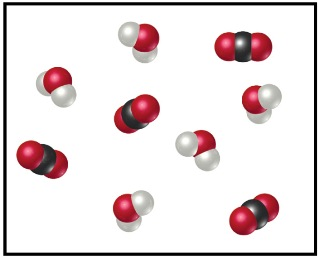# Problem: The following diagram represents the collection of CO2 and H2O molecules formed by complete combustion of a hydrocarbon.What is the empirical formula of the hydrocarbon?

🤓 Based on our data, we think this question is relevant for Professor Shoemaker's class at PSU.

###### FREE Expert Solution

We’re being asked to determine the empirical formula of a hydrocarbon from the combustion diagram above.

Recall that in combustion analysis, a hydrocarbon reacts with excess O2 to form products.

For a compound composed of C ad H the reaction looks like this:

CxHy + O2 (excess) x CO2 + y H2O###### Problem Details
The following diagram represents the collection of CO2 and H2O molecules formed by complete combustion of a hydrocarbon.What is the empirical formula of the hydrocarbon?